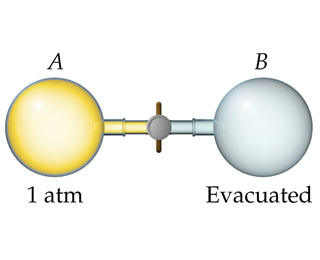# Problem: Consider a system  consisting of the following apparatus, in which gas is confined in one flask and there is a vacuum in the other flask. The flasks are separated by a valve. Assume that the flasks are perfectly insulated and will not allow the flow of heat into or out of the flasks to the surroundings. When the valve is opened, gas flows from the filled flask to the evacuated one.Can you determine the value of E for the process?

###### FREE Expert Solution

We're being asked if we can determine the value of E for the process

Recall that the internal energy of a system is identified with the random, disordered motion of molecules; the total (internal) energy in a system includes potential and kinetic energy.

Recall that the internal energy(ΔE or ΔU) of a system can be calculated from the heat and work of the system. The relationship between internal energy, heat, and work is shown in the following equation:

where

ΔE is the total change in internal energy of a system,

q is the heat exchanged between a system and its surroundings, and

w is the work done by or on the system.

86% (27 ratings)###### Problem Details

Consider a systemconsisting of the following apparatus, in which gas is confined in one flask and there is a vacuum in the other flask. The flasks are separated by a valve. Assume that the flasks are perfectly insulated and will not allow the flow of heat into or out of the flasks to the surroundings. When the valve is opened, gas flows from the filled flask to the evacuated one.

Can you determine the value of E for the process?